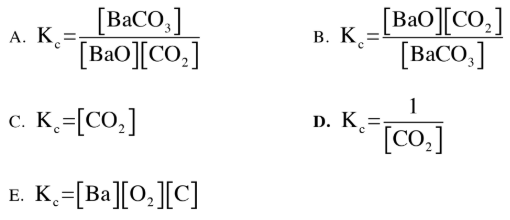# Problem: Which is the correct Kc expression for the following equilibrium reaction?BaO(s) + CO2(g) ⇌ BaCO3(s)

###### FREE Expert Solution

Setup the Kc expression for the reaction and determine which is the correct one for the reaction:

BaO(s) + CO2(g) ⇌ BaCO3(s)

Kc is the equilibrium expression of the concentrations in the reaction. The general form of an equilibrium expression is:###### Problem Details

Which is the correct Kc expression for the following equilibrium reaction?

BaO(s) + CO2(g) ⇌ BaCO3(s)